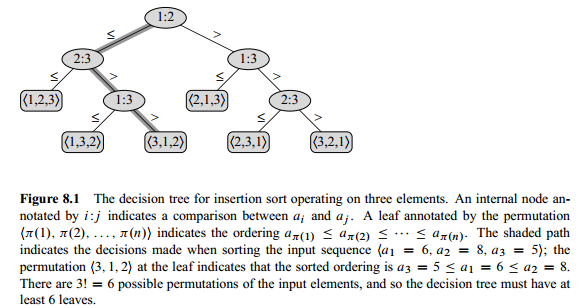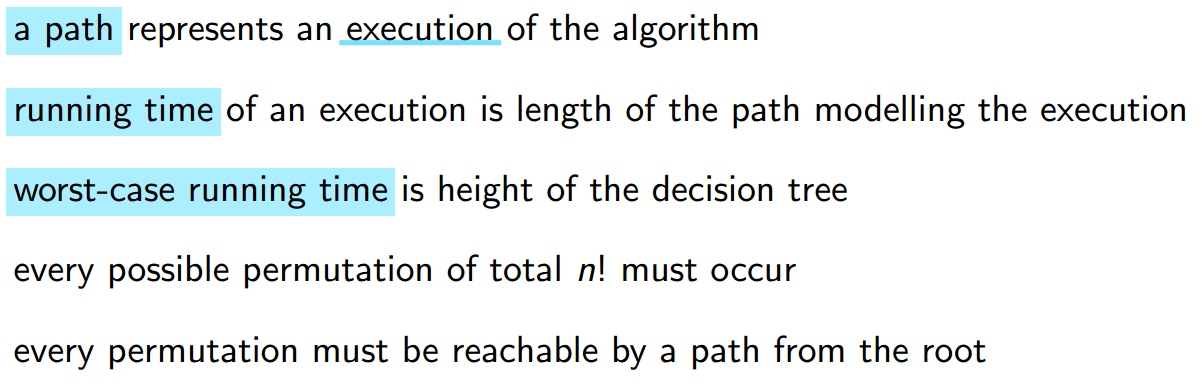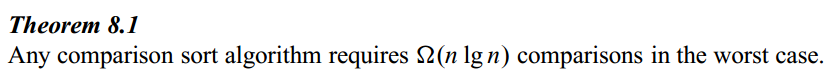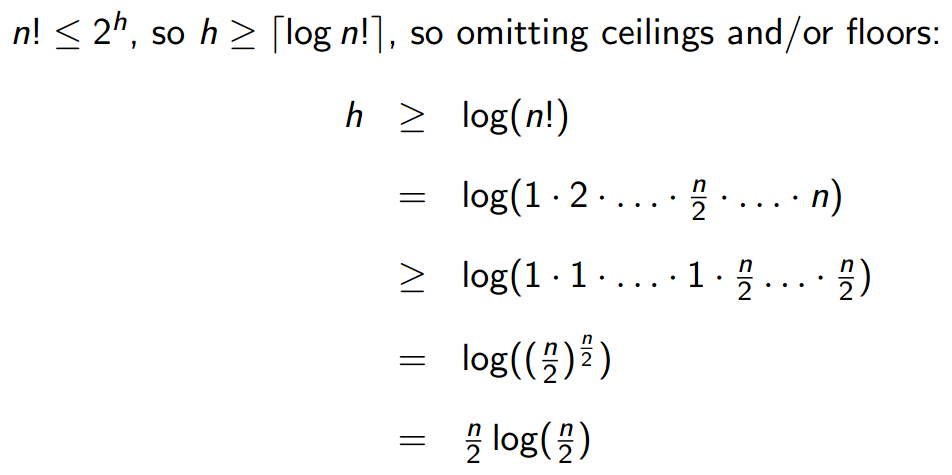# Decision Tree

Updated:

⚠️ This post was created when I was in high school and is no longer maintained.

• In terms of decision trees, we assume without loss of generality that all elements are distinct.
• A decision tree is a full binary tree that represents the comparisons between elements that are performed by a particular sorting algorithm operating on an input of a given size.
• any correct sorting algorithm must be able to produce each permutation of its input, each of the n! permutations on n elements must appear as one of the leaves of the decision tree for a comparison sort to be correct
• Corollary 8.2: Heapsort and merge sort are asymptotically optimal comparison sorts.
—— CLRSTips for this figure:

• The algorithm shown in this figure is insertion sort where we iterate j and i = j - 1• the worst-case comparisons for a comparison algorithm = the height of the decision treeTags: